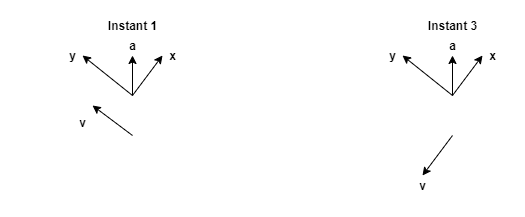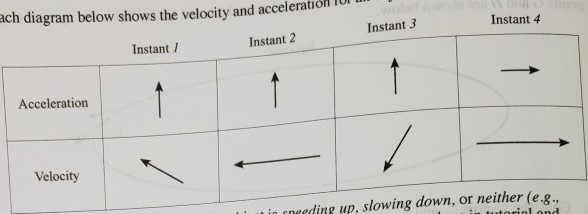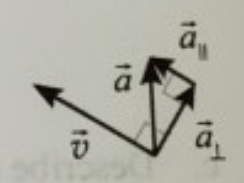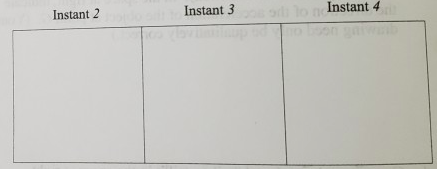# Problem: Each diagram below shows the velocity and acceleration for an object at a certain instant in time.a. For each instant, state whether the object is speeding up, slowing down, or neither (e.g. moving with constant speed). Base your answers on what you have done in tutorial and problem 1.b. The diagram at right illustrates how the acceleration at instant l can be treated as having two components: one parallel to the velocity (a||) and one perpendicular to the velocity (a⊥).For each of the other instants, draw a diagram similar to the one given for instant l. Label the parallel and perpendicular components of the acceleration relative to the velocity. If either component is zero, state so explicitly.c. For each of the instants l-4, compare your descriptions of the motion in part a with the components of the acceleration in part b. Then answer the following:i. Give a general rule that describes how the component of the acceleration parallel to the velocity affects the motion of an object.ii. Give a general rule that describes how the component of the acceleration perpendicular to the velocity affects the motion of an object.

###### FREE Expert Solution

a)

The magnitude of the velocity remains constant only if:

1. a = 0

2. a is perpendicular to v. In this case, only the direction changes. The magnitude remains constant.

For instant 1 and 3, we'll use a tilted coordinate axis.91% (479 ratings)###### Problem Details

Each diagram below shows the velocity and acceleration for an object at a certain instant in time.a. For each instant, state whether the object is speeding up, slowing down, or neither (e.g. moving with constant speed). Base your answers on what you have done in tutorial and problem 1.

b. The diagram at right illustrates how the acceleration at instant l can be treated as having two components: one parallel to the velocity (a||) and one perpendicular to the velocity (a).For each of the other instants, draw a diagram similar to the one given for instant l. Label the parallel and perpendicular components of the acceleration relative to the velocity. If either component is zero, state so explicitly.c. For each of the instants l-4, compare your descriptions of the motion in part a with the components of the acceleration in part b. Then answer the following:
i. Give a general rule that describes how the component of the acceleration parallel to the velocity affects the motion of an object.
ii. Give a general rule that describes how the component of the acceleration perpendicular to the velocity affects the motion of an object.